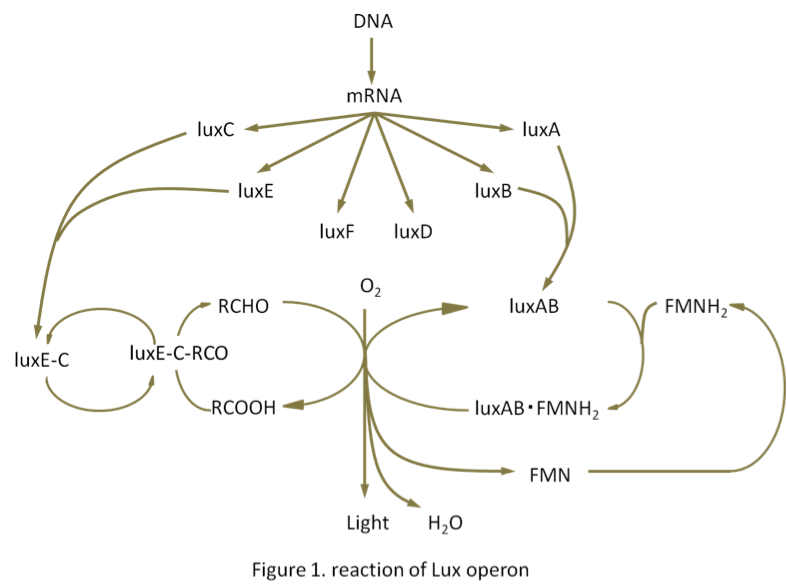# Team:Tokyo-NoKoGen/Project/modelling

Modeling

We aimed to build the mathematical model using MATLAB R2012b SymBiology (http://www.mathworks.com/). However, since we had no knowledge and no sufficient data, so we could not complete the modeling.
For building the mathematical model of lux operon, we investigated about reaction mechanism of lux operon. Figure.1 is described reaction of Lux operon.Primary reaction equations are bellow:

(a) FMNH2 + O2 + RCHO -> FMN + H2O + RCOOH + Light
LuxAB, a luciferase, catalyzes the reaction (a). RCHO is substrate of luciferase (luciferin).